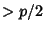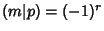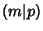## Gauss's Lemma

Let the multiples,, ...,of an Integer such thatbe taken. If there are an Even numberof least Positive Residues modof these numbers, thenis a Quadratic Residue of. Ifis Odd,is a Quadratic Nonresidue. Gauss's lemma can therefore be stated as, whereis the Legendre Symbol. It was proved by Gaußas a step along the way to the Quadratic Reciprocity Theorem.